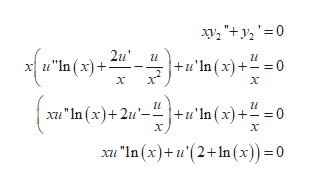# Reduction of order can be used to find the general solution of a non-homogeneous equation.a2(x)y"+a1(x)y'+a0(x)y=g(x)whenever a solution y1 of the associated homogeneous equation is known. In the case of a general homogeneous equation g(x)=0, it turns out this equation can be reduced to a linear first order differential equation by means of a substitution of a non-trivial solution y1.Follow the steps below to use the method of reduction of order to find a second solution y2 to the following differential equation and y1, which solves the given homogeneous equation.xy"+y'=0; y1=ln(x)Let y2=uy1, for u=u(x), and find y2' and y2"Plug y2' and y2" into the differential equation and simplifyUse w=u' to transform the previous answer into a linear first order differential equation in w.Solve for w, and thus u' in the previous answerSolve for u from the previous equation for u'.Solve for y2 using the definition in step 1 (use c1=-1 and c2=0, the constants of integration)Verify that y2 is a solution to the original differential equation, and check that W[y1,y2] does not equal zero on I=all real numbers

Question
74 views

Reduction of order can be used to find the general solution of a non-homogeneous equation.

a2(x)y"+a1(x)y'+a0(x)y=g(x)

whenever a solution y1 of the associated homogeneous equation is known. In the case of a general homogeneous equation g(x)=0, it turns out this equation can be reduced to a linear first order differential equation by means of a substitution of a non-trivial solution y1.

Follow the steps below to use the method of reduction of order to find a second solution y2 to the following differential equation and y1, which solves the given homogeneous equation.

xy"+y'=0; y1=ln(x)

• Let y2=uy1, for u=u(x), and find y2' and y2"
• Plug y2' and y2" into the differential equation and simplify
• Use w=u' to transform the previous answer into a linear first order differential equation in w.
• Solve for w, and thus u' in the previous answer
• Solve for u from the previous equation for u'.
• Solve for y2 using the definition in step 1 (use c1=-1 and c2=0, the constants of integration)
• Verify that y2 is a solution to the original differential equation, and check that W[y1,y2] does not equal zero on I=all real numbers
check_circle

Step 1

Consider the given equation:

Step 2

Now, follow the steps as provided in the question:

Let

Step 3

Now, plug these values in...help_outlineImage Transcriptioncloseху, "+ у, '3D0 х и"In(x)+ 2u" и+u'1n (х) +-3D0 2 х х хи "In (x)+ 2и'- +u'la (x)+— -0 х х "In (x)+ u"(2+1n(х)) %3D0 fullscreen

### Want to see the full answer?

See Solution

#### Want to see this answer and more?

Solutions are written by subject experts who are available 24/7. Questions are typically answered within 1 hour.*

See Solution
*Response times may vary by subject and question.
Tagged in

### Math